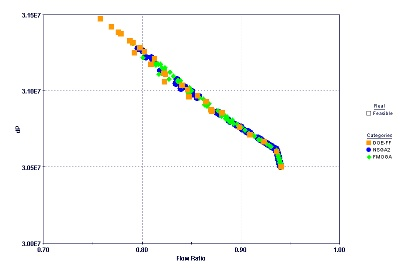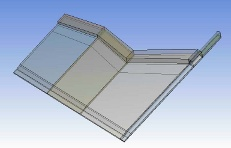# Improving Film Die Flow Uniformity Using Optimization Methods

Author(s):
Patrick C. Lee, Laura Dietsche, Joseph Dooley (The Dow Chemical Company), Sumeet Parashar (ESTECO)

This study shows how the flow uniformity from a film die can be improved by modifying the geometry of the die using a three dimensional finite element optimization technique. In this study, various optimization strategies were used to optimize the geometry to meet the desired objectives of uniform flow at the die exit and minimal pressure drop. Finite element simulations using the numerically optimized geometry predict a more uniform flow than simulations using the baseline geometry. However, some of the numerically optimized die geometries obtained in this study would be impractical to fabricate. Thus we see the power of CFD-based optimization methods to lead towards potentially better performing options; but we also see the necessity of understanding both the die design technology and fabrication techniques in order to analyze the practicality of the proposed optimized solutions.This knowledge can be used to redirect the optimization towards more practical solutions through the use of geometric constraints. In this study, Polyflow, a finite element method (FEM) code and modeFRONTIER, optimization and process integration software, were used to evaluate the effectiveness of an existing coat-hanger die design to distribute polymer melt uniformly across the die. The finite element method is a technique that can be used for numerically solving the mass, momentum, and energy conservation equations for polymer flow.